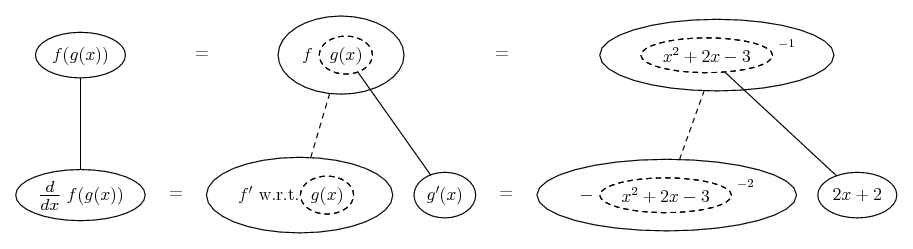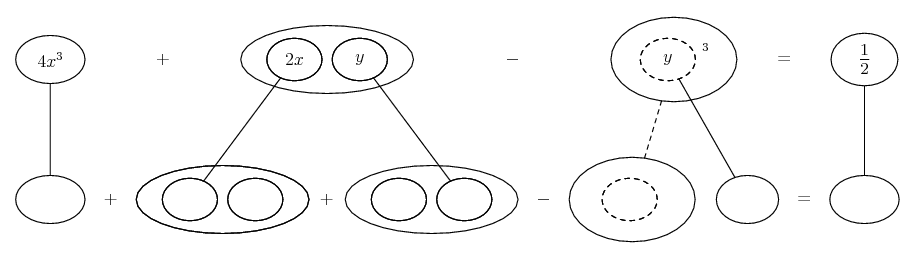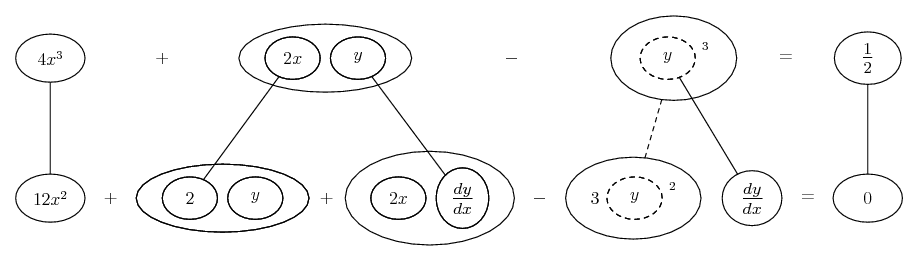# Thread: Need help with a couple calc questions.

1. ## Need help with a couple calc questions.

1.Differentiate: x^2y^3+x = y I got 2x(y^3)+3y^3(x^2)+1=y ... Is that right? I think maybe I did it wrong.

2.The following function may be veiwed as a composite function f(g(x)). Find f(x) and g(x).

1
x^2+2x-3
is f(x)= to 1, and g(x)= to x^2+2x-3? that seems too simple.

3. With this one I'm preatty sure ou need to find the first derivitive, but I don't really know how to go about it.

Assume 4x^3+2xy-y^3=1/2. What is the slope of the graph at the point [1/2,-1]?

-any help would be greatly appreciated thanks.

2.Originally Posted by mike211.Differentiate: x^2y^3+x = y I got 2x(y^3)+3y^3(x^2)+1=y ... Is that right? I think maybe I did it wrong.

-any help would be greatly appreciated thanks.
$\displaystyle x^2y^3+x = y$

$\displaystyle 2x(y^3)+3y^2y'(x^2)+1=y'$

3.Originally Posted by mike212.The following function may be veiwed as a composite function f(g(x)). Find f(x) and g(x).

1
x^2+2x-3
is f(x)= to 1, and g(x)= to x^2+2x-3? that seems too simple.
If that's meant to be 1 over the rest, and by "= to 1" you mean reciprocal i.e. f(x) = 1/x or x to power minus one, then no, it's correct and not too simple.

Edit:

Just in case a picture helps...3. With this one I'm preatty sure ou need to find the first derivitive, but I don't really know how to go about it.

Assume 4x^3+2xy-y^3=1/2. What is the slope of the graph at the point [1/2,-1]?
-any help would be greatly appreciated thanks.
As with the first, differentiate throughout (not forgetting the chain rule), then plug in the x and y values for the given point. I'll put a pic.

Edit:... where... is the product rule, and...... the chain rule. Straight continuous lines differentiate downwards (integrate up) with respect to x, and the straight dashed line similarly but with respect to the dashed balloon expression (the inner function of the composite which is subject to the chain rule).

Spoiler:Substitute into the bottom row...

$\displaystyle 12(\frac{1}{2})^2\ +\ 2(-1)\ +\ 2(\frac{1}{2})\frac{dy}{dx}\ -\ 3(-1)^2\ \frac{dy}{dx}\ =\ 0$

and solve to find dy/dx

_________________________________________

Don't integrate - balloontegrate!

Balloon Calculus; standard integrals, derivatives and methods

Balloon Calculus Drawing with LaTeX and Asymptote!

4. you said to differentiate through the function and plug in the x and y values, but what do I differentiate in respect to? x or y.

5. Good point - unless otherwise indicated, assume y is differentiable with respect to x and apply the chain rule accordingly. This is called implicit differentiation because we don't know y in terms of x.

#### Search Tags

calc, couple, questions